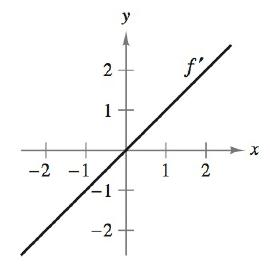Chapter 5.1, Problem 41E### Calculus: An Applied Approach (Min...

10th Edition
Ron Larson
ISBN: 9781305860919

#### Solutions

Chapter
Section### Calculus: An Applied Approach (Min...

10th Edition
Ron Larson
ISBN: 9781305860919
Textbook Problem
3 views

# Interpreting a Graph In Exercises 39–42, the graph of the derivative of a function is given. Sketch the graphs of two functions that have the given derivative. (There is more than one correct answer.)To determine

To graph: The two functions of the derivative whose graph is shown by,Explanation

Given Information:

The graph of the provided derivative is shown below.

Graph:

From the graph, there is a straight line passes through origin and for positive value of x the corresponding value of derivative function is positive while for negative values of x the corresponding value of derivative function is negative.

So, the derivative function is given as,

f(x)=x

Integrate the above derivative function use the basic rule of integration xndx=xn+1n+1+C.

f(x)dx=xdxf(x)=x1+11+1+C=x22+C

The value of C is any constant. It can by any numerical value.

Substitute 0 for C in above function.

f(x)=x22+0=x22

Substitute 2 for C in above function.

f(x)=x22+2

Therefore, the function for that derivative is (f(x)=x) are f(x)=x22 or f(x)=x22+2.

Graph the function f(x)=x22. It is quadratic function so the graph of this function is parabola.

Now, choose some values for x and compute the value for f(x), in order to form the ordered pairs that will plot on the graph.

So, put x=2 in the function f(x)=x22.

f(2)=(2)22=42=2

Now, put x=0 in the function f(x)=x22,

f(0)=(0)22=0

Now, put x=2 in the function f(x)=x22,

f(2)=(2)22=42=2

The required table is shown below,

 x f(x) (x,f(x)) −2 2 (−2,2) 0 0 (0,0) 2 2 (2,2)

Therefore, the graph of the function f(x)=x22 as shown below,

### Still sussing out bartleby?

Check out a sample textbook solution.

See a sample solution

#### The Solution to Your Study Problems

Bartleby provides explanations to thousands of textbook problems written by our experts, many with advanced degrees!

Get Started

#### Equations Solve the equation graphically. 140. x+4=x25

Precalculus: Mathematics for Calculus (Standalone Book)

#### In problems 63-73, factor each expression completely. 70.

Mathematical Applications for the Management, Life, and Social Sciences

#### For y = (x3 + 1)2, y = _____. a) 2x(x3 + 1) b) 6x(x3 + 1) c) 6x2(x3 + 1) d) 3x2(x3 + 1)

Study Guide for Stewart's Single Variable Calculus: Early Transcendentals, 8th